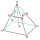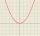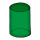# Volume + quadratic equation - math problems

#### Number of problems found: 37

• The surfaceThe surface of the cylinder is 1570 cm2, its height is 15 cm. Find its volume and radius of the base.
• Truncated pyramidThe truncated regular quadrilateral pyramid has a volume of 74 cm3, a height v = 6 cm, and an area of the lower base 15 cm2 greater than the upper base's content. Calculate the area of the upper base.
• The blockThe block, the edges formed by three consecutive GP members, has a surface area of 112 cm2. The sum of the edges that pass through one vertex is 14 cm. Calculate the volume of this block.
• ConsiderConsider all square prisms with a height of 10 cm. If x is the measurement of the base edge, in cm, and y is the volume of the prism, in cm3. Graph the function
• The cylinderIn a rotating cylinder it is given: the surface of the shell (without bases) S = 96 cm2 and the volume V = 192 cm cubic. Calculate the radius and height of this cylinder.
• Rotary cylinderIn the rotary cylinder it is given: surface S = 96 cm2 and volume V = 192 cm cubic. Calculate its radius and height.
• Hard cone problemThe surface of the cone is 200 cm², its height is 7 centimeters. Calculate the volume of this cone.
• Consecutive membersThe block has a volume of 1728 cm³. Determine the lengths of the edges a, b, c of the blocks for which a < b < c and a + b + c = 38 cm and whose numerical values in cm represent three consecutive members of the geometric sequence.
• The poolThe cube-shaped pool has 140 cubic meters of water. Determine the dimensions of the bottom if the depth of the water is 200 cm and one dimension of the base is 3 m greater than the other. What are the dimensions of the pool bottom?
• Shell area cyThe cylinder has a shell content of 300 cm square, while the height of the cylinder is 12 cm. Calculate the volume of this cylinder.
• The cylinderThe cylinder has a surface area of 300 square meters, while the cylinder's height is 12 m. Calculate the volume of this cylinder.
• Magnified cubeIf the lengths of the cube's edges are extended by 5 cm, its volume will increase by 485 cm3. Determine the surface of both the original and the magnified cube.
• Block or cuboidThe wall diagonals of the block have sizes of √29cm, √34cm, √13cm. Calculate the surface and volume of the block.
• Conical bottleWhen a conical bottle rests on its flat base, the water in the bottle is 8 cm from its vertex. When the same conical bottle is turned upside down, the water level is 2 cm from its base. What is the height of the bottle?
• Uboid volumeCalculate the cuboid volume if the walls are 30cm², 35cm², 42cm²
• Secret treasureScouts have a tent in the shape of a regular quadrilateral pyramid with a side of the base 4 m and a height of 3 m. Find the container's radius r (and height h) so that they can hide the largest possible treasure.
• Faces diagonalsIf a cuboid's diagonals are x, y, and z (wall diagonals or three faces), then find the cuboid volume. Solve for x=1.3, y=1, z=1.2
• Two pipesHow long will the pool be filled with a double supply pipe if it takes the pool to fill the first pipe by 4 hours longer and the second pipe 9 hours longer than both pipes open at the same time?
• Cuboid wallsIf the areas of three adjacent faces of a cuboid are 8 cm², 18 cm² and 25 cm². Find the volume of the cuboid.
• Solid cuboidA solid cuboid has a volume of 40 cm3. The cuboid has a total surface area of 100 cm squared. One edge of the cuboid has a length of 2 cm. Find the length of a diagonal of the cuboid. Give your answer correct to 3 sig. Fig.

Do you have an exciting math question or word problem that you can't solve? Ask a question or post a math problem, and we can try to solve it.

We will send a solution to your e-mail address. Solved examples are also published here. Please enter the e-mail correctly and check whether you don't have a full mailbox.

Looking for help with calculating roots of a quadratic equation? Tip: Our volume units converter will help you with the conversion of volume units. Volume - math problems. Quadratic Equations Problems.